# Measuring Instruments

Subjects:

• General
• Analog meter
• Digital multimeter
• Resolution
• Set measuring range
• Calculating Absolute Error
• Calculate Relative Error
• Multimeter measuring
• Oscilloscope measuring

General:
A lot is measured in the techniques. On this page, measuring with regard to automotive technology is discussed. In automotive technology, measurements can be taken in many different ways, namely during development, testing, monitoring processes and finding faults. When one knows how to measure, all that is needed is literature (current flow diagrams) to determine where to measure.

The most commonly used (electrical) measuring equipment in automotive technology are:

• The multimeter / analog meter: It is used to measure voltage (U), current (I) and resistance (R). The digital multimeter will show the value in the LCD screen, and the analog meter will indicate the measured value on an underlying scale using a needle.
• The oscilloscope: The oscilloscope measures voltages that can be recorded in a timeline. This timeline can be set (number of Volts on the Y-axis and the time course on the X-axis).

Analog gauge:
The analog meter (moving coil meter) consists of a permanent magnet and a moving coil. The current flowing through the moving coil creates a magnetic field. The forces that the magnetic field exert on each other cause the moving coil (with the pointer mounted on it) to rotate. The greater the current (and thus the magnetic field) the further the pointer will swing.

Advantages compared to the digital multimeter:

• Cheap;
• More accurate below 10 Hz (not above that anymore).

Cons:

• Relatively slow due to the moving pointer.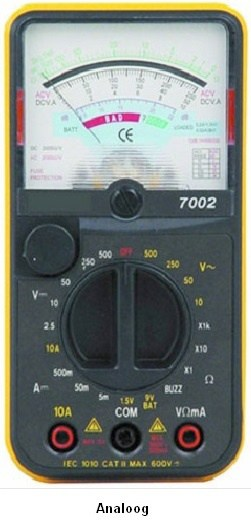Digital multimeter:
The digital multimeter is a replacement for the analog meter. The meters are being further developed (in accuracy, speed and functions). The multimeter contains an A/D converter. The analog signal being measured is first processed before being displayed. This operation depends on the selected function (volts, amps, ohms, etc.). The digitized signal is then sent to the display. The speed at which this happens is called the “response time”, which can be found in the specifications of the meter. The response time (of the A/D converter) is the time it takes to register a change in the input signal. The more expensive the meter is, the lower this response time will be.

There are digital multimeters with a manual and an automatic range setting. Sets the measuring range. The multimeter in the picture below does this automatically. The chapter “Measuring range” is described further on this page.Resolution:
The number of digits displayed by multimeters determines the resolution and thus also the reading accuracy of the meter. The resolution therefore only has to do with the screen and not with the measuring range. There are 3½, 3¾ and 4½ digit multimeters. The more digits the multimeter can display, the more numbers are possible (so a more accurate measurement).

3½ digits:
This is a standard multimeter, which can measure up to 200 V accurately in the 0,1 V range. If a reading was taken where the actual voltage would be 22,66 V, the meter would read 22,6 V.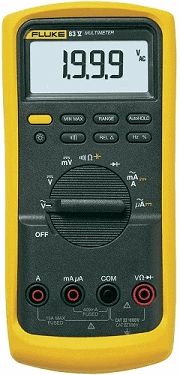3¾ digits:
With this multimeter the resolution has increased by a factor of 10 and with the same measurement (of 22,66 V with the 3½ digit multimeter) it will actually indicate 22,66 V. That is one hundredth of a Volt more (and therefore more accurate).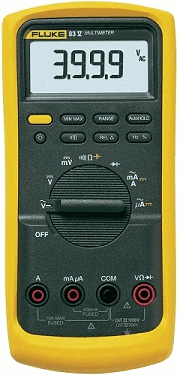4½ digits:
This multimeter has an extra digit available in all ranges. The resolution has again increased by a factor of 10.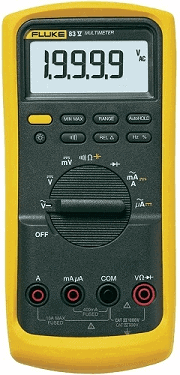Set measuring range:
The measuring range of the multimeter below can be set manually. This is necessary to obtain the most accurate result possible with each measurement. When measuring the battery voltage, it is best to choose the option 20 DCV. For example, the battery voltage will be displayed as 12.41. It is best to select a measuring range that will be below the maximum measuring result. For example, the battery voltage will never exceed 99 volts. If a larger resolution was chosen (of 200 DCV), the battery voltage would be indicated as 12.4 (less accurate). This has to do with the resolution:

 Range: Resolution: 200 mV 0,1 mV 2 V 0,001V 20 V 0,01 V 200 V 0,1 V 2000 V 1 V

Examples of this table:

• When measuring a voltage of 100 Volts in the 200 V range, the meter will read 100,1 V. When this same voltage is measured in the 2000 V range, the meter will read 100 V (less accurate).
• When measuring a voltage of 9,188 Volts in the 2 V range, the meter will read 9,188 V. When this same voltage is measured in the 200 V range, the meter will indicate 9,2 V (rounded, so less accurate).

The most accurate measurement therefore depends on which measuring range is set and on the resolution of the screen. With low resolution screens, the most accurate voltage cannot be displayed with an accurate measuring range.

With the multimeter shown, the measuring range can only be adjusted manually. The more extensive multimeters have an "Autorange" button where the meter itself sets the best measuring range (based on its own resolution). Only with simple multimeters can only be selected for the position Volt, Ampere (etc.) and the measuring range is often 20 V as standard (so with a resolution of 0,01 V).
Another problem is that there is always a deviation in the meter. When setting a resolution that is too low, the deviation is greatest. More about this in the next chapters “Absolute and Relative Errors” further down the page.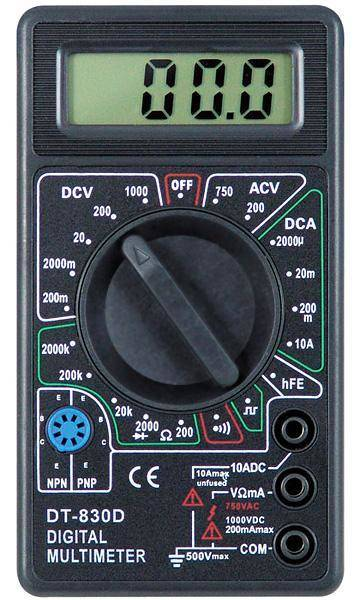Calculating Absolute Error:
Every multimeter has a certain accuracy. This accuracy can be found in the specifications (in the manual). With this data the deviation of the measurement can be calculated. 2 concepts can be calculated; the “absolute error” and the “relative error”. The absolute error is the voltage in Volts and the relative error is calculated in percent.

Example:
Voltage (U) = 12,55 V
±(0,3%rdg + 1d)
rdg = reading = the value read on the display (the measured value)
1d = 1 digit = the resolution (on the 20 V range, 1 digit corresponds to 0,01 V and on the 2 V range to 0,001 V).

The actual voltage is 12,55 Volts. This is measured on the 20 V range.
0,3% rdg is 0,3% of 12,55 V = 0,038 V.
On the 20 V range, 1d = 0,01 V.

The total absolute error is then: the reading + 1 digit = Absolute error. In numbers: 0,038 + 0,01 = 0,048 V

The final answer with the absolute error is:
U = 12,55 ± 0,05 V.
That means that the measurement is somewhere between 12,50 and 12,60 Volts.

Cheap multimeters often have a larger deviation than the more expensive, so that the total absolute error is also greater. This now proves that “cheap multimeters” cannot take accurate measurements.

Calculating Relative Error:
When the absolute error is calculated as a percentage of the reading, it is called the relative error. This relative error is usually used when comparing the meters.

The relative error on the previous multimeter is: total absolute error / (divide by) the actual voltage x (multiply by) 100% = the relative error.
In numbers: U = 0,038 / 12,55 x 100 = 0,30%.

The final answer with the relative error is:
U = 12,55 ± 0,3%.

12,55 V minus the 0,3% gives the answer 12,50. Plus 0,3% is then 12,60. This is the same as what was calculated with the absolute error, but stated in percentages.

Measuring with the multimeter:
Voltage, current, and resistance are all measured differently. How to measure properly with the multimeter is explained with examples on the page measure with the multimeter.

Measuring with the Oscilloscope:
An oscilloscope (abbreviated scope) is a graphic voltmeter. The voltage is displayed graphically as a function of time. The scope is also very accurate. The time can be set so small that signals from sensors such as the oxygen sensor or actuators such as an injector can be reproduced perfectly.

How to measure with the scope is explained on the page measure with the oscilloscope.error: Alert: Content is protected !!